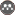Volume 13, Issue 49 (Water and Soil Science 2009)                   JWSS 2009, 13(49): 167-177 | Back to browse issues page

BibTeX | RIS | EndNote | Medlars | ProCite | Reference Manager | RefWorks
Send citation to:Ghanavati N, Malakouti M, Hossein por A. Correlation of Q/I Parameters with Some Soil Properties and Potassium Uptake by Wheat in Some Soils of Abyak Region. JWSS. 2009; 13 (49) :167-177
URL: http://jstnar.iut.ac.ir/article-1-984-en.html
, navid_989@yahoo.com
Abstract:   (28725 Views)
Correlation between components of Q/I and wheat (Triticum aestivum L.) growth indices was studied in a greenhouse experiment during 2003-04 growing season. Eighty soil samples (0 – 30 cm depth) were collected randomly in farms around the Abyak region, Ghazvin province. Twenty-one samples were then selected based on soil texture and NH4OAC-K. The research included treatments of 21 soils and two potassium (K) levels (0, 100 mg/kg) and was conducted in a factorial manner in a randomized complete block design with three replications for studying correlations between Q/I and treatments. Effect of potassium application on the wheat growth was found to be significant at 5% level. The effect of soil properties on wheat growth was also found to be significant at 1% level. However, the interaction of K and soil was not significant. There were no significant differences among the different equilibrium time intervals. The soil potassium buffering capacity (PBCK) values were strongly correlated with CEC (r =0.996**), clay content (r = 0.921**) and moisture saturation percentage (r = 0.811**). Final equation derived from stepwise regression for prediction of PBCK was as follows: PBCK = 7.419 CEC –19.743 R2adj = 0.782** The equilibrium potassium activity ratios (ARke) were strongly correlated with soluble potassium (r =0.846**), NH4OAC-K (r =0.730**), SP (r = 0/794*), OC (r = -0.477ns) and clay content (r=-0.602*). Similarly, readily exchangeable potassium (ΔK0) was strongly correlated with ammonium acetate extractable potassium (r = 0.871**), soluble potassium (r = 0.778**), saturation percentage (r = 0.551*), organic carbon percentage (r=-0.045ns) and clay content (r=-0.206ns). There was no significant correlation between ΔK0 and ARKe with potassium uptake values by wheat. In contrast, there was a strong correlation between PBCK values and potassium uptake by wheat (r = 0.729**), and relative wheat yield (r = 0.735**). There was no significant correlation between Q/I parameters when KCl and K2SO4 were used, and the soil physicochemical properties. However, Q/I parameters obtained from KCl showed a higher correlation with wheat plant's growth indices.